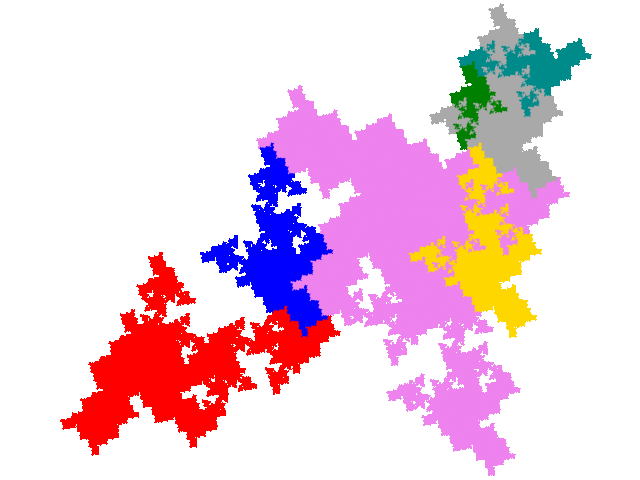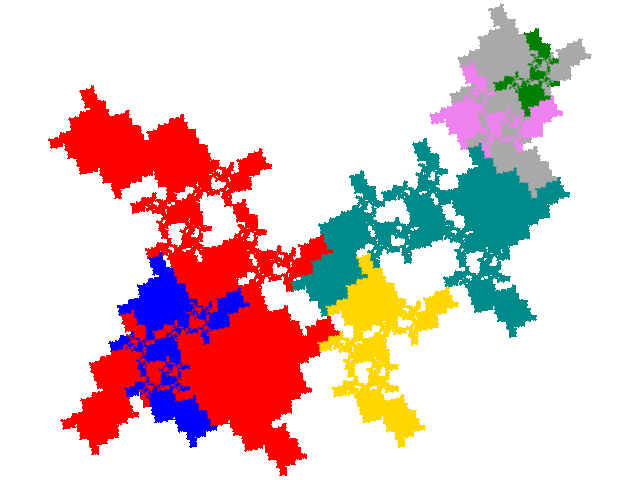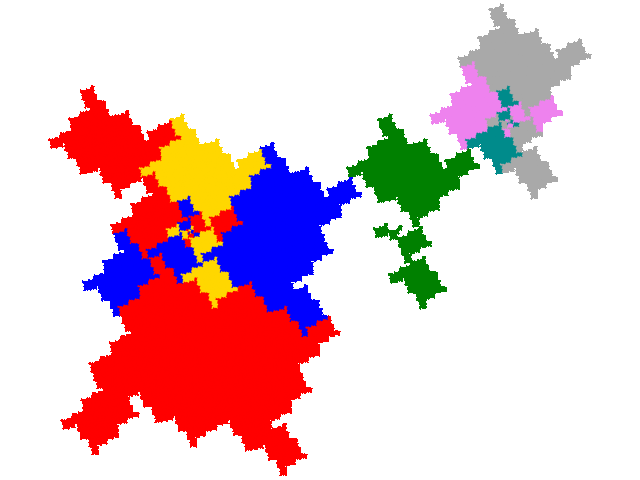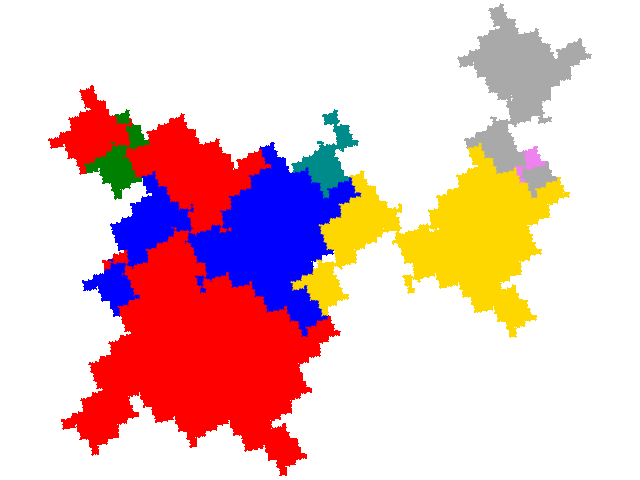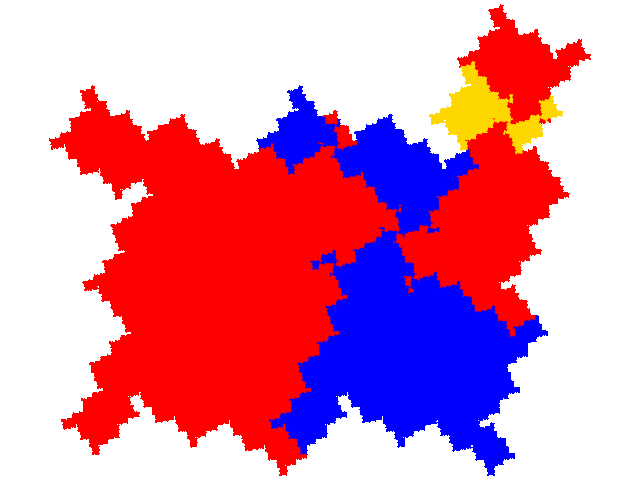# Some tiles associated with the 6th unit cubic Pisot number

## Alloexternal Tiles

As each order 3 tile has 9 potential legitimate order 7 partial post(allo)composition derivatives and there are two order 3 “external” tiles there are a potential 18 order 7 “alloexternal” tiles. However 9 of the attractors are disconnected, reducing the number to 9.

For the first “external” tile there are 3 derived tiles. One is a simple teragon with dissection equation c + c2 + 3c3 + c4 + c5 = 1 and tiling signature 012.The other two are complex teragons, also with dissection equation c + c2 + 3c3 + c4 + c5 = 1 and tiling signature 012.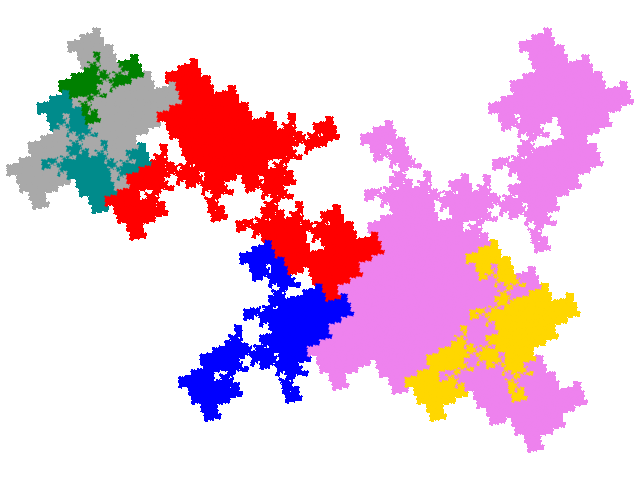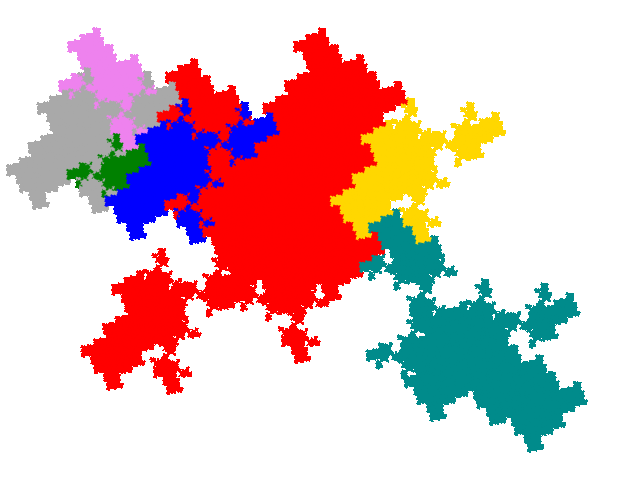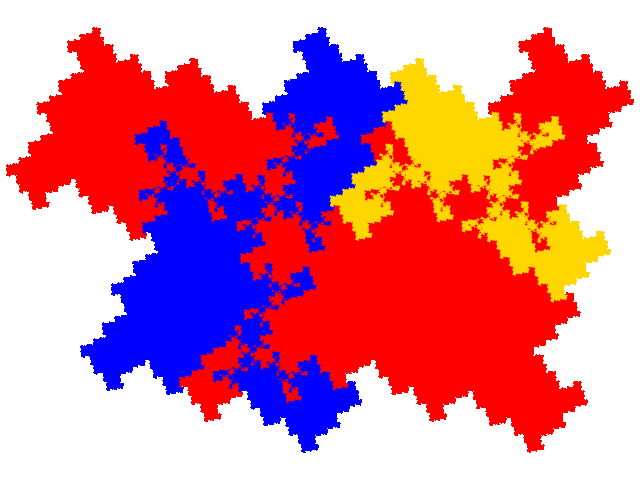The other “external” tile gives rise to 6 derived tiles. There are two simple teragons, one with dissection equation c + c2 + 3c3 + c4 + c5 = 1 and tiling signature 012, and the other with disssection equation 2c + 2c4 + 2c7 + c9 = 1 and tiling signature 036.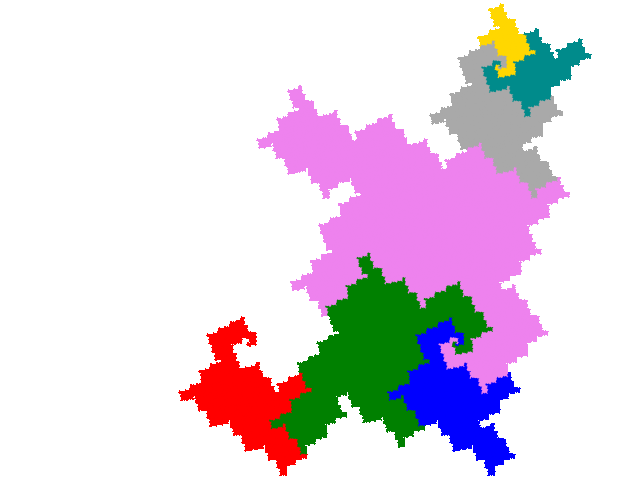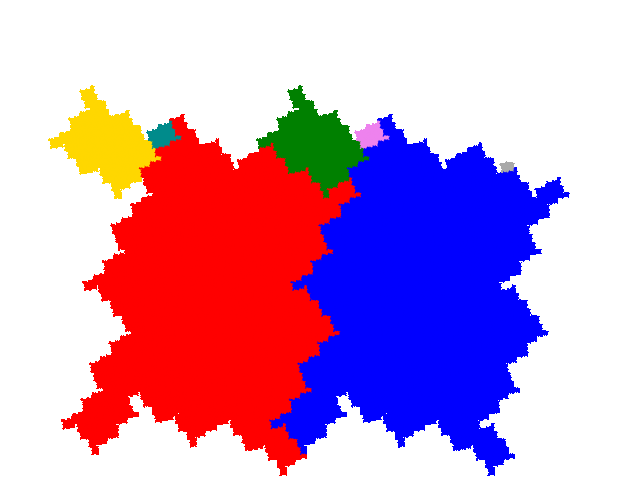There are four complex teragons. Three have dissection equation c + c2 + 3c3 + c4 + c5 = 1 and tiling signature 012, and the other with dissection equation c + 2c2 + c3 + 2c5 + c7 = 1 and tiling signature 014.